### Home > CCG > Chapter 5 > Lesson 5.3.5 > Problem5-133

5-133.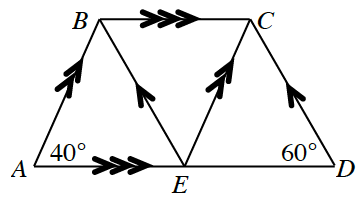Examine trapezoid $ABCD$ below.

1. Find the measures of all the angles in the diagram.

The corresponding angles are equal.

Since line $\overline{AB}$ $\Vert$ line $\overline{EC}$, then corresponding angles $∠BAE=∠CED$.
So $∠CED=40º$, mark it on your diagram.
While line $\overline{CD}$ $\Vert$ line $\overline{EB}$, then corresponding angles $∠CDE=∠BEA$.
So $∠BEA=60º$, mark it on your diagram.

Use the Triangle Angle Sum Theorem.

Now you know two angles in $ΔABE$ and $ΔCED$, knowing that one angle is $40º$ and $60º$.
Calculate the third angle using the equation $40º+60º+\text{missing angle}=180º$.
So the missing angle is $80º$. Mark it in your diagram.

The angles at E form a straight angle, which is $180º$.

Since $∠BEA=60º$ and $∠CED=40º$ then $40º+60º+∠CEB=180º$.
Solve for $∠CEB$, mark it on your diagram. $∠CEB=80^{\circ}$.

The alternate interior angles are equal.

Since $∠BEA=60º$ and $∠CED=40º$, then $∠EBC=60º$ and $∠ECB=40º$ because alternate angles are equal. Mark the angles on your diagram.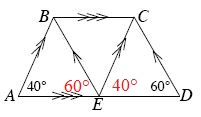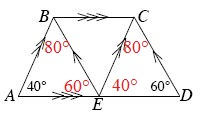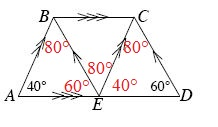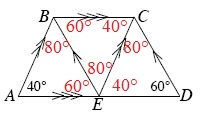2. What is the sum of the angles that make up the trapezoid $ABCD$? That is, what is $m∠A+m∠ABC+m∠BCD+m∠D$?

$40º+\left(80º+60º\right)+\left(40º+80º\right)+60º=360º$
Note: Do not add in the angles at $E$.